# GCSE Maths – Percentages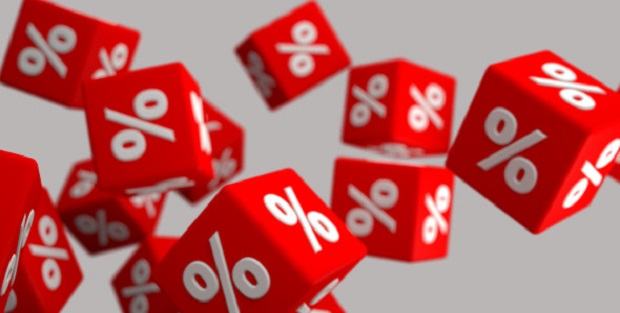A percentage is a standardised form for a fraction, in which it is quoted as a fraction of 100. To change a percentage into a decimal or a fraction we divide it by 100%; to change a decimal or a fraction into a percentage we multiply it by 100%.

Examples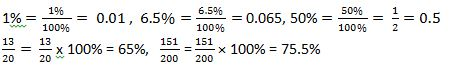To get a percentage of a number, multiply the number by the percentage expressed as a fraction or a decimal.

Examples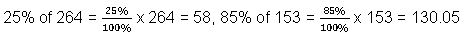To express one number as a percentage of another, write the two numbers as a fraction and multiply by 100%.

Example

Express 117 as a percentage of 500: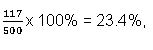To calculate a percentage change (either increase or decrease) use the formula

Percentage change =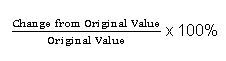[NB: A very common mistake is to use the new value instead of the original value]

Example

A company had expected sales of £15,000 last month, but when the actual sales for the month were totalled they came to £17,280. By what percentage did the actual sales exceed the expected?

Increase = £17,280 – £15,000 = £2,280, so percentage increase =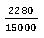× 100% = 15.2%

### Work Towards That 'A' Grade

Achieve the results you want with-written revision resources when you register with iRevise.
Its Free!